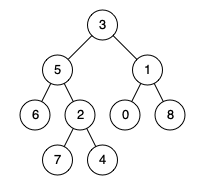Given a binary tree, find the lowest common ancestor (LCA) of two given nodes in the tree. According to wikipedia,

The lowest common ancestor is defined between two nodes p and q as the lowest node in T that has both p and q as descendants (where we allow a node to be a descendant of itself).

For example, we have a binary tree like this:Given node 5 and node 1, returns 3.
Given node 5 and node 4, returns 5. (node 5 is the ancestor of itself)

The solution is provided as below:

``````    public TreeNode lowestCommonAncestor(TreeNode root, TreeNode node1, TreeNode node2) {

if (root == null || root == node1 || root == node2) {
return root;
}

// Divide
TreeNode left = lowestCommonAncestor(root.left, node1, node2);
TreeNode right = lowestCommonAncestor(root.right, node1, node2);

// Conquer
if (left != null && right != null) {
return root;
}
if (left != null) {
return left;
}
if (right != null) {
return right;
}
return null;
}

``````

The idea is that if there is LCA, then we return LCA (left and right not equal to null). Otherwise, return left node if left node is not null or right node if right node is not null. If none of the nodes are found, then return null.

The hardest part of this question to identify the exit condition. Here, we can clearly see that we return when root is null, or the root is equal to either first or second node.

#### What if we need to find LCA of Binary Search Tree (BST)?

We can make use of the attributes of BST data structure which parent node value is greater than left node value but smaller than right node value.

``````    public TreeNode lowestCommonAncestor(TreeNode root, TreeNode node1, TreeNode node2) {
if(root.val > node1.val && root.val > node2.val){
return lowestCommonAncestor(root.left, node1, node2);
}else if(root.val < node1.val && root.val < node2.val){
return lowestCommonAncestor(root.right, node1, node2);
}else{
return root;
}
}
``````

Because in a BST, we can guarantee that if the root value is greater than both node1 and node2, then the ancestor must be in the root's left node. If the root value is smaller than both node1 and node2, then the ancestor must be in the root's right node. If none of the above is valid, then simply return the root node will do.

That's it!

Post was published on , last updated on .

Like the content? Support the author by paypal.me!